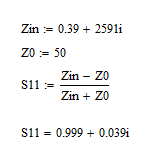[SOLVED]Normalize to fixed impedance 50 Ohm. CST

Status
Not open for further replies.

Fovakis

Full Member level 1Is anyone here can help me what this means??

What is the differencec with or without it?

thanks a lot :evil:

V

volker_muehlhaus

GuestThis is related to the Z0 problem in your other post.

For wave guide ports, some 3D simulators define Z0 as line impedance at the port ("generalized S-parameters"). This means that any straight line will have perfect match, no matter what the dimensions are. However, the simulator does know that Z0 value and can re-normalize the S-parameters to 50 ohm port impedance. This is what the switch does: it allows you to switch between generalized S-parameters and 50 Ohm S-parameters.

Fovakis

Full Member level 1I almost understand what you mean my friend, but i need an example.

Icase_Without normalize to 50Ohm, for e=2 and some specific dimensions, a waveguide port gives |s11|=0,40 phase=93degrees and |Zin|=|0.29+i2591|=2591Ohm with phase=89 ,also VSWR=2,31.

IIcase_With normalize to 50Ohm the same one is::lol: |s11|=0,94 with phase=14degrees, and |Zin|=|0.39+i2591|=2591 ohm and phase=89, also VSWR=36,13 8-O.

As we can see the Zin impedance of the waveguide did't change cause i didn't changed any of the materials and the dimensions.

But the S11 changed much and i don't understand it.The formula s11=Zin-Zo/Zin+Z0 for s11,Zin complex numbers

is it right for each case?

Thanks for all i apreciate thisV

volker_muehlhaus

GuestAs we can see the Zin impedance of the waveguide did't change cause i didn't changed any of the materials and the dimensions.

Yes, exactly.

If you now calculate the S11 value for your chosen reference impedance Z0, that S11=(Zin-Z0)/(Zin+Z0) will depend on the value of Z0.

Fovakis

Full Member level 1Yes, exactly.

If you now calculate the S11 value for your chosen reference impedance Z0, that S11=(Zin-Z0)/(Zin+Z0) will depend on the value of Z0.

Can you please explain mathematicaly the numbers of s11,phase,Zin and Zo that i have write above?:evil:

V

volker_muehlhaus

GuestFovakis

Full Member level 1hehe it is not that i am boring bro. It is that the numbers with "normalize to 50" ,for |s11|=0,94 with phase=14degrees, and |Zin|=|0.39+i2591|=2591 ohm and phase=89 ARE WRONG. If you take these numbers and search for Zo you will not have 50. That's my question . WHY?

V

volker_muehlhaus

GuestOk, I see what you mean.You should contact CST support to find out.

Fovakis

Full Member level 1I will go ask my professor at the university...i he don't know i will ask support team! Thanks a lot for all!erasteHello,

Between the Z and S matrix the relation is not as simple as you write. You can not write that S11 = (Z11-Zo)/(Z11+Zo). Have a look at a HF book to see the relations. In fact you find that :
Z11 = Zo ((1+S11)(1-S22)+S12*S21)/((1-S11)(1-S22)-S12*S21))
And so on for the others parameters.

•Fovakis

Fovakis

Points: 2

Fovakis

Full Member level 1ok...so...the exression Z11 where is refering to? I understand at the resistance of the waveguide at the port I, right?!

V

volker_muehlhaus

Guest@eraste: You are correct for Z11, but this is not what we discussed. Zin is the input impedance into port 1 with the other ports terminated.

Fovakis

Full Member level 1@Volker. Yes but CST produces Z11,Z21 not Zin :O! The Zin how do we calculated from Cst? All the above at my posts that i have write about Zin are reffering for Z11.

So if the formula of eraste is correct for the determination of Z11 we need not only s11, but s21,s22,s12 !!!

volker are you running Cst or HFSS or both?

Thanks for the company broV

volker_muehlhaus

Guestvolker are you running Cst or HFSS or both?

None one them. I use Sonnet (planar) and Empire XCcell (3D FDTD).

erasteThe waveguide is seen like "quadrupole" . Between the S matrix (S11 S12 S21 S22) and the Z matrix (Z11 Z12 Z21 Z22) , we have not the relation Z11 = Zo (1+S11)/(1-S11).
Refer at any book deal with filter or scatering parameters. This latest relation is true only for "dipole".

Fovakis

Full Member level 1@eraste!can you make any attachment of the notes you have about it?

So for my restrangular waveguide Z11 = Zo ((1+S11)(1-S22)+S12*S21)/((1-S11)(1-S22)-S12*S21))

is ok?>!

eraste•Fovakis

Fovakis

Points: 2

Fovakis

Full Member level 1Thanks so much eraste !!!!

Status
Not open for further replies.